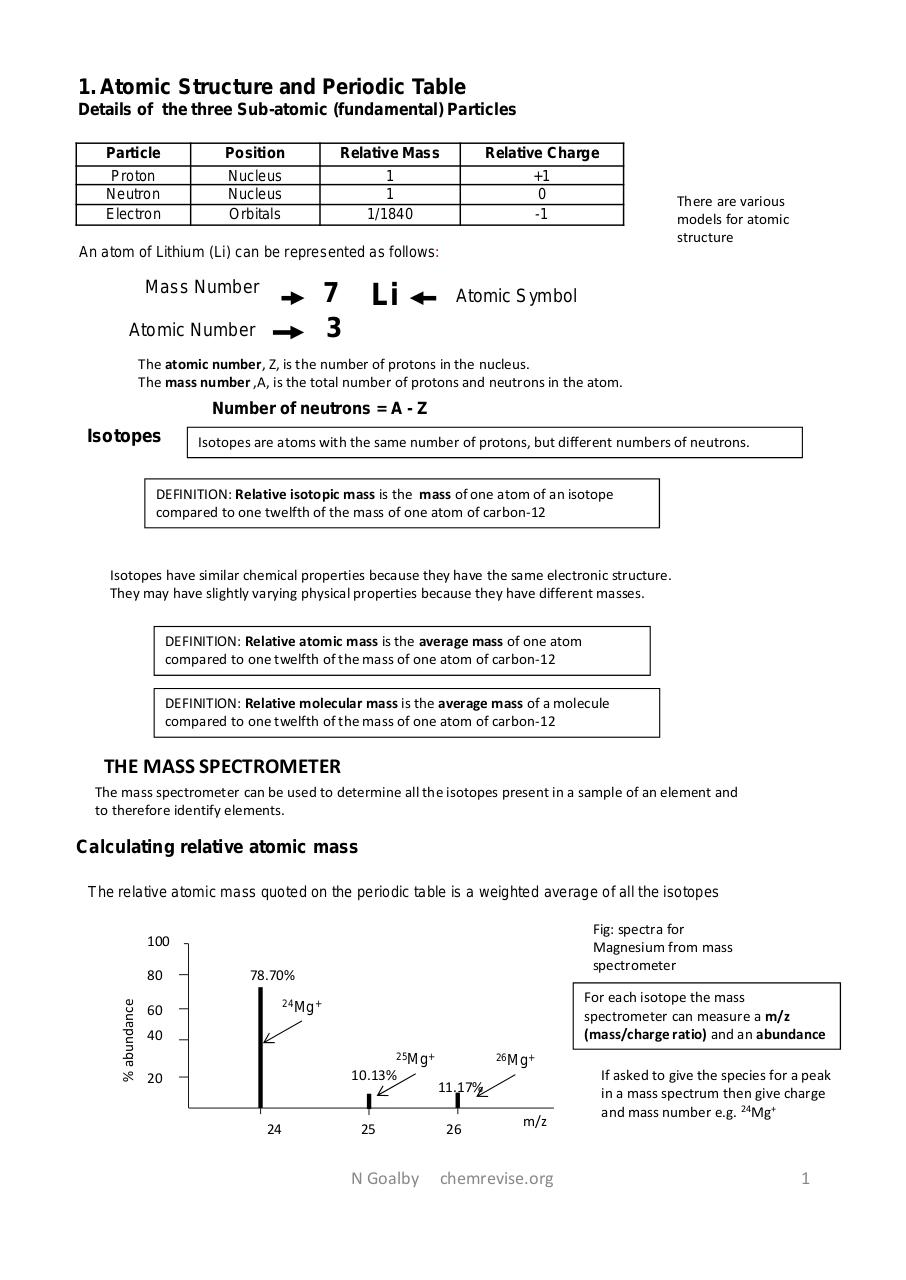# chemistry full notes.pdfPage 123184

#### Text preview

1. Atomic Structure and Periodic Table
Details of the three Sub-atomic (fundamental) Particles
Particle

Position

Relative Mass

Relative Charge

Proton
Neutron
Electron

Nucleus
Nucleus
Orbitals

1
1
1/1840

+1
0
-1

There are various
models for atomic
structure

An atom of Lithium (Li) can be represented as follows:

Mass Number

7
3

Atomic Number

Li

Atomic Symbol

The atomic number, Z, is the number of protons in the nucleus.
The mass number ,A, is the total number of protons and neutrons in the atom.

Number of neutrons = A - Z

Isotopes

Isotopes are atoms with the same number of protons, but different numbers of neutrons.

DEFINITION: Relative isotopic mass is the mass of one atom of an isotope
compared to one twelfth of the mass of one atom of carbon-12

Isotopes have similar chemical properties because they have the same electronic structure.
They may have slightly varying physical properties because they have different masses.
DEFINITION: Relative atomic mass is the average mass of one atom
compared to one twelfth of the mass of one atom of carbon-12
DEFINITION: Relative molecular mass is the average mass of a molecule
compared to one twelfth of the mass of one atom of carbon-12

THE MASS SPECTROMETER
The mass spectrometer can be used to determine all the isotopes present in a sample of an element and
to therefore identify elements.

Calculating relative atomic mass
The relative atomic mass quoted on the periodic table is a weighted average of all the isotopes
Fig: spectra for
Magnesium from mass
spectrometer

100

% abundance

80

78.70%

60

For each isotope the mass
spectrometer can measure a m/z
(mass/charge ratio) and an abundance

24Mg+

40
25Mg+

10.13%

20
24

25

N Goalby

26Mg+

11.17%
26

m/z

chemrevise.org

If asked to give the species for a peak
in a mass spectrum then give charge
and mass number e.g. 24Mg+

1